# Scientific NotationPage 1

#### WATCH ALL SLIDES

Slide 1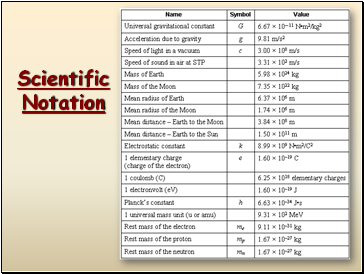## Scientific Notation

Slide 2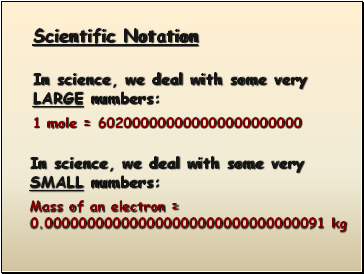In science, we deal with some very LARGE numbers:

1 mole = 602000000000000000000000

In science, we deal with some very SMALL numbers:

Mass of an electron =

0.000000000000000000000000000000091 kg

Scientific Notation

Slide 3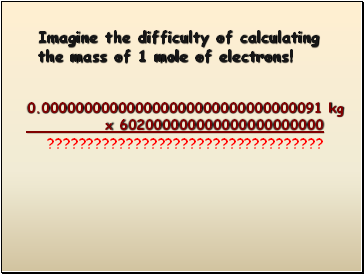Imagine the difficulty of calculating the mass of 1 mole of electrons!

0.000000000000000000000000000000091 kg

x 602000000000000000000000

???????????????????????????????????

Slide 4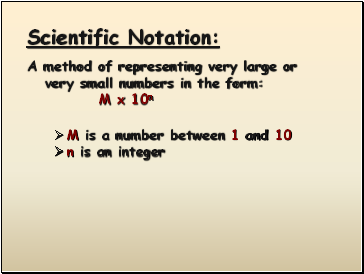Scientific Notation:

A method of representing very large or very small numbers in the form:

M x 10n

M is a number between 1 and 10

n is an integer

Slide 5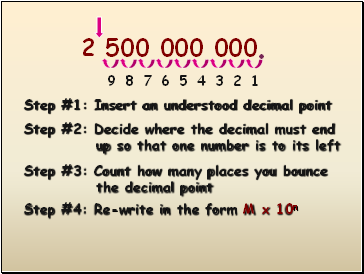2 500 000 000

Step #1: Insert an understood decimal point

.

Step #2: Decide where the decimal must end

up so that one number is to its left

Step #3: Count how many places you bounce

the decimal point

1

2

3

4

5

6

7

8

9

Step #4: Re-write in the form M x 10n

Slide 6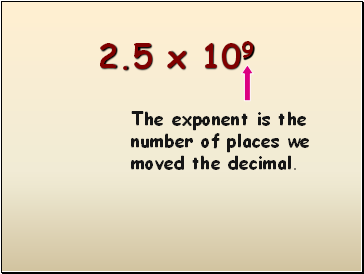2.5 x 109

The exponent is the number of places we moved the decimal.

Slide 7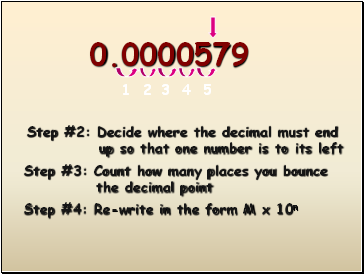0.0000579

Step #2: Decide where the decimal must end

up so that one number is to its left

Step #3: Count how many places you bounce

the decimal point

Step #4: Re-write in the form M x 10n

1

2

3

4

5

Slide 8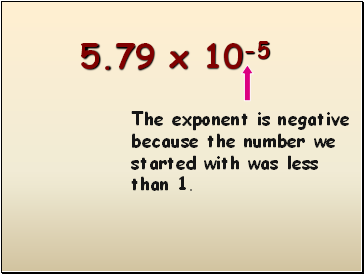5.79 x 10-5

The exponent is negative because the number we started with was less than 1.

Slide 9## Performing calculations in scientific notation

Slide 10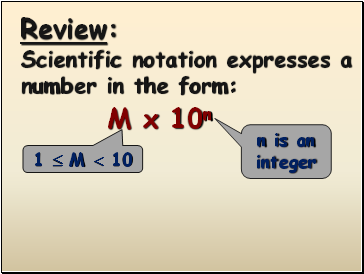Review:

Scientific notation expresses a number in the form:

M x 10n

1  M  10

n is an integer

Slide 114 x 106

+ 3 x 106

IF the exponents are the same, we simply add or subtract the numbers in front and bring the exponent down unchanged.

7

x 106

Slide 12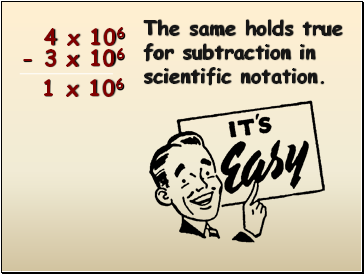4 x 106

- 3 x 106

The same holds true for subtraction in scientific notation.

Go to page:
1  2